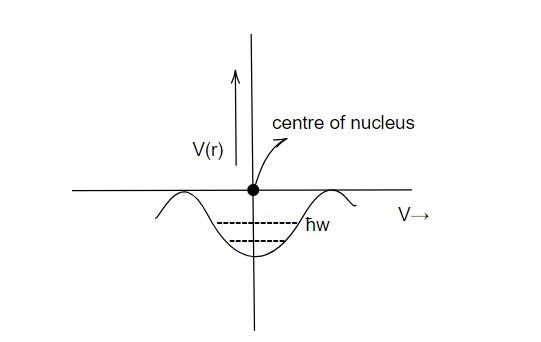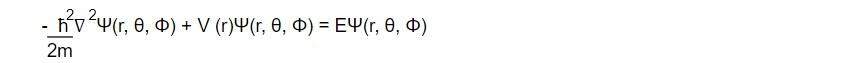# Shell model

In nuclear physics, the shell model is a theoretical model used to explain the properties of atomic nuclei. The model is based on the idea that nucleons (protons and neutrons) occupy energy levels, or "shells," in a similar way to electrons in an atom. The energy levels are determined by the strong nuclear force, which holds the protons and neutrons together in the nucleus.

The basic idea of the shell model is that the nucleons occupy different energy levels in the nucleus, similar to the way electrons occupy different energy levels in an atom. The nucleons are grouped into shells, and each shell is characterized by a specific energy level. The lowest energy level is called the "ground state," and higher energy levels are called "excited states."

In the shell model, protons and neutrons are treated as independent particles, each with their own set of energy levels. The number of nucleons in each shell is determined by the nuclear spin and the parity of the nucleon.

In shell model, the nucleons are assumed to move in different closed shells just like electrons which revolve round the nucleus in different atomic shells or orbits. Each nucleon is also assumed to move independently under the effect of centrally symmetric potential created due to average interaction among remaining (A-1) nucleons. The nuclear shells get closed for certain number of protons and neutron number. The number of neutrons or protons in nuclear states are also governed by Pauli exclusion principle.

Evidences in favor of shell model or magic number

1. The nuclei having both protons or neutrons number as magic number are found to be most abundant and stable in nature.

2. The nuclei with magic number of protons or neutrons has peak in Binding energy curve.

3. The nuclei with protons number as magic number has higher number of isotopes.

4. The nuclei with magic number of neutrons have higher number of isotones. For example: N= 50 has 5 isotones and N=82 has 7 isotones.

5. The radioactive series Uranium, Therium, Achnium, Neptunium decay which have both protons and neutrons as magic number.

6. The energy of β particle is maximum if the product nucleus in decay has magic number of protons of neutrons.

7. The binding energy of neutron or proton next to magic number has lower value.

8. The magic number nuclei has zero electric quadrupole moment which indicates more stability due to spherical charge distribution.

9. The capturing cross section of magic number of nuclei is very low.

Assumption of shell model of nucleus

1. Each nucleon is assumed to move in different nuclear shells as do the electrons in atomic orbits.

2. Each nucleon move independently under the effect of centrally symmetric potential produced due to average interaction among remaining A-1 nucleons where A = total number of nucleons.

3. Each nuclear shell gets closed when it is occupied by certain number of neutrons and protons. The number of neutrons and protons in the nuclear shells are as guided by Pauli's exclusion principle.

4. Each nucleon is assumed to have spin angular momentum.

Harmonic oscillator potentialThe different nuclear energy states and their energy are obtained by solving Schrondinger wave equation for the nucleon of mass (m) and potential as that of harmonic oscillator.

The Schrodinger wave equation becomeswhere Ψ(r, θ, Φ) is wave function as a function of spherical polar coordinates (r, θ, Φ) and E is energy Eigen value.

The solution of above equation can be obtained as

where n = principle quantum number

l= orbital quantum number

m= magnetic quantum number

Eλ = (λ + 3/2) ħw ...............1

where λ = (2n+ l -2) .............2

where λ = harmonic oscillator quantum number

n = 1,2,3,.....

l = 0,1,2.......(n-1)

λ =0, Eo = 3/2 ħw

λ = 1, E1 = 5/2 ħw

from eq1, it is seen that the energy states are separated by the energy ħw and the energy are quantized.

The number of degenerate levels (levels having same energy) for any l is given as

2 (2l +1) -> occupation number

The energy states for nucleons for any value l are represented by the same spectroscopic notation for the electron i.e for l =0,1,2,3,4,5.......etc, the energy states that any value of λ can accommodate = (λ+1)(λ+2)

So cumulative number of nucleons upto any λ = 1/3 (λ+1)(λ+2)(λ+3)

The consideration of harmonic oscillator potential successfully explains the magic number 28,20. Since, the nuclear state get fulfilled if they are occupied by the nucleons number 2,8,20. So, the nuclei with those number of nucleons are most stable. but other magic number found evidently are 28,50,82,126 which are not explained by this model.

Achievement of shell model:

1. The shell model successfully explains the existence of magic number and the stability of nucleus having magic number of protons and neutrons.

2. It can explain the ground state properties like parity, nuclear spin, magnetic moment and electric quadrupole moment.

This note is taken from Nuclear physics, BSC physics, Nepal.

This note is a part of the Physics Repository.